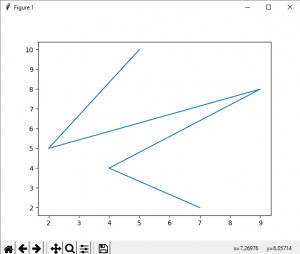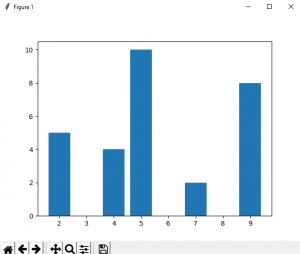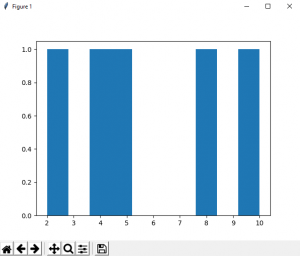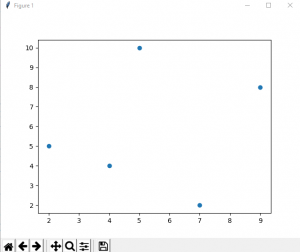# Introduction to Matplotlib

## Python | Introduction to Matplotlib

Matplotlib is an amazing visualization library in Python for 2D plots of arrays. Matplotlib is a multi-platform data visualization library built on NumPy arrays and designed to work with the broader SciPy stack. It was introduced by John Hunter in the year 2002.

One of the greatest benefits of visualization is that it allows us visual access to huge amounts of data in easily digestible visuals. Matplotlib consists of several plots like line, bar, scatter, histogram etc.

Installation :
Windows, Linux and macOS distributions have matplotlib and most of its dependencies as wheel packages. Run the following command to install `matplotlib `package :

`python -mpip install -U matplotlib`

Importing matplotlib :

``````from matplotlib import pyplot as plt
or
import matplotlib.pyplot as plt ``````

#### Basic plots in Matplotlib :

Matplotlib comes with a wide variety of plots. Plots helps to understand trends, patterns, and to make correlations. They’re typically instruments for reasoning about quantitative information. Some of the sample plots are covered here.

Line plot :

 `# importing matplotlib module ` `from` `matplotlib ``import` `pyplot as plt` ` ` `# x-axis values` `x ``=` `[``5``, ``2``, ``9``, ``4``, ``7``]` ` ` `# Y-axis values` `y ``=` `[``10``, ``5``, ``8``, ``4``, ``2``]` ` ` `# Function to plot` `plt.plot(x,y)` ` ` `# function to show the plot` `plt.show()`

Output :Bar plot :

 `# importing matplotlib module ` `from` `matplotlib ``import` `pyplot as plt` ` ` `# x-axis values` `x ``=` `[``5``, ``2``, ``9``, ``4``, ``7``]` ` ` `# Y-axis values` `y ``=` `[``10``, ``5``, ``8``, ``4``, ``2``]` ` ` `# Function to plot the bar` `plt.bar(x,y)` ` ` `# function to show the plot` `plt.show()`

Output :Histogram :

 `# importing matplotlib module ` `from` `matplotlib ``import` `pyplot as plt` ` ` `# Y-axis values` `y ``=` `[``10``, ``5``, ``8``, ``4``, ``2``]` ` ` `# Function to plot histogram` `plt.hist(y)` ` ` `# Function to show the plot` `plt.show()`

Output :Scatter Plot :

 `# importing matplotlib module ` `from` `matplotlib ``import` `pyplot as plt` ` ` `# x-axis values` `x ``=` `[``5``, ``2``, ``9``, ``4``, ``7``]` ` ` `# Y-axis values` `y ``=` `[``10``, ``5``, ``8``, ``4``, ``2``]` ` ` `# Function to plot scatter` `plt.scatter(x, y)` ` ` `# function to show the plot` `plt.show()`

Output :Reference : Matplotlib Documentation.

Last Updated on March 1, 2022 by admin

## Play sound in PythonPlay sound in Python

Play sound in Python In this article, we will see how to play sound in

## Deploy Python Flask App on HerokuDeploy Python Flask App on Heroku

Deploy Python Flask App on Heroku Flask is a web application framework written in Python.

## numpy.random.randn() in Pythonnumpy.random.randn() in Python

numpy.random.randn() in Python The numpy.random.randn() function creates an array of specified shape and fills it with random

## sys.stdout.write in Pythonsys.stdout.write in Python

sys.stdout.write in Python This is a built-in Python module that contains parameters specific to the

## Python program to get all subsets of given size of a setPython program to get all subsets of given size of a set

Python program to get all subsets of given size of a set Given a set,

## How to change the Tkinter label text?How to change the Tkinter label text?

How to change the Tkinter label text?   Tkinter is a standard GUI (Graphical user interface)

## Python – Get list of running processesPython – Get list of running processes

Python – Get list of running processes A Process is a program that is being executed (processed).